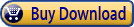Summary: Want to access your data indirectly? The answer is to use Excel's lookup function. ExcelTips: Powerful Lookup Functions is a key resource in discovering how to use those functions. Here is the complete table of contents for this resource.ExcelTips: Powerful Lookup Functions, fourth edition, includes 131 information-packed pages that will help you discover and master the power behind the lookup functions provided by Excel. The following is the Table of Contents:

## Introduction

• Macros and Array Formulas
• Need More Tips?
• Sharing this Document

## Lookup Function Basics

• Understanding the CHOOSE Function
• Understanding the MATCH Function
• Understanding the INDEX Function
• Understanding the OFFSET Function
• Understanding the VLOOKUP Function
• Understanding the HLOOKUP Function
• Understanding the LOOKUP Function
• Maintaining Text Formatting in a Lookup
• Errors when Copying References to External Cells

• Cell Address of a Maximum Value
• Finding the Address of the Lowest Value in a Range
• Referring to the Last Cell
• Referencing the Last Cell in a Column
• Relative References within Named Ranges
• Stopping a Formula from Updating References
• Incrementing References by Multiples when Copying Formulas
• Where Is that Text?

## Finding Duplicate Values or Unique Values

• Synchronizing Lists
• Comparing Lists for Duplicates
• Cleaning Up Lists
• Ensuring Unique Values in a Column
• Counting Unique Values
• Getting a Count of Unique Names
• Finding Differences Between Lists
• Getting Rid of Everything Except Numbers
• Deleting All Names but a Few

## Grabbing Info from a List

• Returning a Weight and a Date
• Retrieving the Last Value in a Column
• Last Non-Zero Value in a Row
• Extracting Numbers within a Range
• Highlighting Cells Containing both Letters and Numbers
• Determining a State from an Area Code
• Figuring Out the Low-Score Winner
• Determining Winners, by Category
• Determining "Highest Since" or "Lowest Since"
• Returning Blanks or Asterisks from a Lookup
• Returning Item Codes Instead of Item Names
• Looking Backward through a Data Table
• Pulling a Phone Number with a Known First and Last Name
• Looking Up Names when Key Values are Identical
• Reversing Integer Values
• Compiling a List of Students in a Course
• Determining a Name for a Week Number
• Complex Lookup Formulas

## Using Lookup Functions with Dates

• Macro for Month Name
• Automatically Advancing by a Month
• Adding Ordinal Notation to Dates
• Date for Next Wednesday
• Calculating Future Workdays
• Calculating the First Business Day of the Month
• Determining Business Quarters from Dates
• Specifying Different Weekends with NETWORKDAYS
• Finding the Dates for Minimums and Maximums
• Deciphering a Coded Date
• Finding the Date Associated with a Negative Value

## Looking Up Random Data

• Picking Different Random Numbers from a Range
• Selecting Random Names
• Generating Random Strings of Characters

## Calculations Involving Lookups

• Calculating the Interval between Occurrences
• Inserting Dashes between Letters and Numbers
• Breaking Up Variable-Length Part Numbers
• Finding the First Non-Digit in a Text Value
• Calculating the Median Age of a Group of People
• Determining a Simple Moving Average
• Averaging the Last Numbers in a Column
• Referencing the Last Six Items in a Formula
• Engineering Calculations

## Adjusting the Way VLOOKUP Works

• Making VLOOKUP Trigger a Macro
• Making VLOOKUP Case Sensitive
• Returning Blanks with VLOOKUP
• Using VLOOKUP to Access Information to the Left
• Concatenating Values from a Variable Number of Cells

## Using Lookup Functions to Enhance Charts

• Easily Changing Chart Data Ranges
• Automatically Updating Charts for Additional Data
• Automatically Creating Charts for Individual Rows in a Data Table
• Creating a Year-to-Date Comparison Chart

ExcelTips: Powerful Lookup Functions